Advertisement Remove all ads

# Geometry Shaalaa.com Model Set 5 2019-2020 SSC (English Medium) 10th Standard Board Exam Question Paper Solution

Advertisement Remove all ads
Geometry [Shaalaa.com Model Set 5]
Marks: 40Academic Year: 2019-2020
Date: March 2020
Duration: 2h

1
1.A | MCQs
1.A.i

Some question and their alternative answer are given. Select the correct alternative.

Find perimeter of a square if its diagonal is $10\sqrt{2}$

10 cm

$40\sqrt{2}$cm

20 cm

40 cm

Concept: Apollonius Theorem
Chapter: [0.02] Pythagoras Theorem
1.A.ii

Choose the correct alternative answer for the following question.

1 + tan2 $\theta$  = ?

cot2θ

cosec2θ

sec2θ

tan2θ

Concept: Application of Trigonometry
Chapter: [0.06] Trigonometry
1.A.iii

Four alternative answers for the following question is given. Choose the correct alternative.
Chords AB and CD of a circle intersect inside the circle at point E. If AE = 5.6, EB = 10, CE = 8, find ED.

7

8

11.2

9

Concept: Touching Circles
Chapter: [0.03] Circle
1.A.iv

Fill in the blank using correct alternative.

Out of the following, point ........ lies to the right of the origin on X– axis.

(–2,0)

(0,2)

(2,3)

(2,0)

Concept: Slope of a Line
Chapter: [0.04] Co-ordinate Geometry
1.B
1.B.i

Some question and their alternative answer are given. Select the correct alternative.

In ∆ABC, AB = $6\sqrt{3}$ cm, AC = 12 cm, BC = 6 cm. Find measure of ∠A.

30°

60°

90°

45°

Concept: Apollonius Theorem
Chapter: [0.02] Pythagoras Theorem
1.B.ii

Prove the following.

tan4θ + tan2θ = sec4θ - sec2θ

Concept: Trigonometric Ratios of Complementary Angles
Chapter: [0.06] Trigonometry
1.B.iii

The radii of ends of a frustum are 14 cm and 6 cm respectively and its height is 6 cm. Find its  curved surface area

Concept: Frustum of a Cone
Chapter: [0.07] Mensuration
1.B.iv

The radius of a circle is 10 cm. The measure of an arc of the circle is 54°. Find the area of the sector associated with the arc. ($\pi$= 3.14 )

Concept: Length of an Arc
Chapter: [0.07] Mensuration
2
Advertisement Remove all ads
2.A | Solve any 2 of the following
2.A.i

In ∆ABC, AB = 10, AC = 7, BC = 9 then find the length of the median drawn from point C to side AB

Concept: Right-angled Triangles and Pythagoras Property
Chapter: [0.02] Pythagoras Theorem
2.A.ii

In the given figure, in a circle with centre O, length of chord AB is equal to the radius of the circle. Find measure of each of the following.
(1) ∠ AOB (2)∠ ACB
(3) arc AB (4) arc ACB.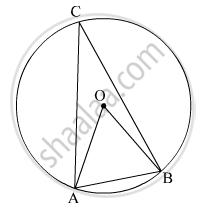Concept: Property of Sum of Measures of Arcs
Chapter: [0.03] Circle
2.A.iii

In the given figure, ∆QRS is an equilateral triangle. Prove that,
(1) arc RS ≅ arc QS ≅ arc QR
(2) m(arc QRS) = 240°.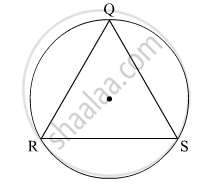Concept: Property of Sum of Measures of Arcs
Chapter: [0.03] Circle
2.B | Solve any 4 of the following
2.B.i

Draw a circle of radius 3.6 cm. Draw a tangent to the circle at any point on it without using the centre.

Concept: Construction of Tangent Without Using Centre
Chapter: [0.05] Geometric Constructions
2.B.ii

Construct ∆PYQ such that, PY = 6.3 cm, YQ = 7.2 cm, PQ = 5.8 cm. If $\frac{YZ}{YQ} = \frac{6}{5},$ then construct ∆XYZ similar to ∆PYQ.

Concept: Division of a Line Segment
Chapter: [0.04] Co-ordinate Geometry [0.05] Geometric Constructions
2.B.iii

Show that the points A(1, 2), B(1, 6), $C\left( 1 + 2\sqrt{3}, 4 \right)$ are vertices of an equilateral triangle.

Concept: Standard Forms of Equation of a Line
Chapter: [0.04] Co-ordinate Geometry
2.B.iv

Two buildings are in front of each other on a road of width 15 meters. From the top of the first building, having a height of 12 meter, the angle of elevation of the top of the second building is 30°.What is the height of the second building?

Concept: Heights and Distances
Chapter: [0.06] Trigonometry
2.B.v

In the given figure, if A(P-ABC) = 154 cm2  radius of the circle is 14 cm, find

(1) ∠APC

(2) l ( arc ABC) .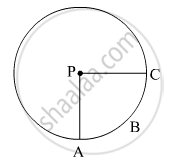Concept: Circumference of a Circle
Chapter: [0.07] Mensuration [0.07] Mensuration
3
3.A | Solve any 1 of the following
Advertisement Remove all ads
3.A.i

In adjoining figure, seg PS ⊥ seg RQ seg QT ⊥ seg PR. If RQ = 6, PS = 6 and PR = 12, then Find QT.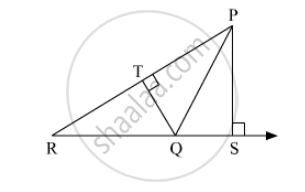Concept: Similar Triangles
Chapter: [0.01] Similarity
3.A.ii

Walls of two buildings on either side of a street are parellel to each other. A ladder 5.8 m long is placed on the street such that its top just reaches the window of a building at the height of 4 m. On turning the ladder over to the other side of the street , its top touches the window of the other building at a height 4.2 m. Find the width of the street.

Concept: Right-angled Triangles and Pythagoras Property
Chapter: [0.02] Pythagoras Theorem
3.B | Solve any 2 of the following
3.B.i

In trapezium PQRS, side PQ || side SR, AR = 5AP, AS = 5AQ then prove that, SR = 5PQ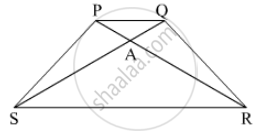Concept: Properties of Ratios of Areas of Two Triangles
Chapter: [0.01] Similarity
3.B.ii

Draw a circle with centre O and radius 3.5 cm. Take point P at a distance 5.7 cm from the centre. Draw tangents to the circle from point P.

Concept: Construction of Tangent to the Circle from the Point on the Circle
Chapter: [0.05] Geometric Constructions
3.B.iii

Prove the following trigonometric identities.

1/(sec A + tan A) - 1/cos A = 1/cos A - 1/(sec A - tan A)

Concept: Trigonometric Identities
Chapter: [0.06] Trigonometry
3.B.iv

In the given figure, BC is a tangent to the circle with centre O. OE bisects AP. Prove that ΔAEO~Δ ABC.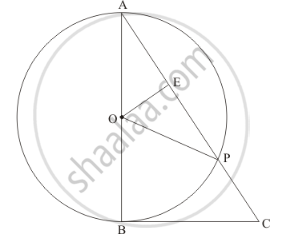Concept: Concept of Circle - Centre, Radius, Diameter, Arc, Sector, Chord, Segment, Semicircle, Circumference, Interior and Exterior, Concentric Circles
Chapter: [0.03] Circle
4 | Solve any 2 of the following
4.A

The areas of two similar triangles are 121 cm2 and 64 cm2 respectively. If the median of the first triangle is 12.1 cm, find the corresponding median of the other.

Concept: Areas of Similar Triangles
Chapter: [0.01] Similarity
4.B

In the given figure, OQ : PQ = 3.4 and perimeter of Δ POQ = 60 cm. Determine PQ, QR and OP.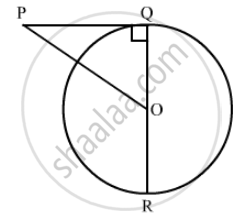Concept: Concept of Circle - Centre, Radius, Diameter, Arc, Sector, Chord, Segment, Semicircle, Circumference, Interior and Exterior, Concentric Circles
Chapter: [0.03] Circle
4.C

Two vertices of an isosceles triangle are (2, 0) and (2, 5). Find the third vertex if the length of the equal sides is 3.

Concept: Coordinate Geometry
Chapter: [0.04] Co-ordinate Geometry
5 | Solve any 1 of the following
Advertisement Remove all ads
5.A

In the given figure,

$\square$ PQRS is a rectangle. If PQ = 14 cm, QR = 21 cm, find the areas of the parts x, y, and z.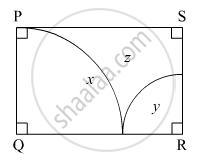Concept: Circumference of a Circle
Chapter: [0.07] Mensuration [0.07] Mensuration
5.B

Some plastic balls of radius 1 cm were melted and cast into a tube. The thickness, length and outer radius of the tube were 2 cm, 90 cm, and 30 cm respectively. How many balls were melted to make the tube?

Concept: Concept of Surface Area, Volume, and Capacity
Chapter: [0.07] Mensuration
Advertisement Remove all ads

#### Request Question Paper

If you dont find a question paper, kindly write to us

View All Requests

#### Submit Question Paper

Help us maintain new question papers on Shaalaa.com, so we can continue to help students

only jpg, png and pdf files

## Maharashtra State Board previous year question papers 10th Standard Board Exam Geometry with solutions 2019 - 2020

Maharashtra State Board 10th Standard Board Exam Geometry question paper solution is key to score more marks in final exams. Students who have used our past year paper solution have significantly improved in speed and boosted their confidence to solve any question in the examination. Our Maharashtra State Board 10th Standard Board Exam Geometry question paper 2020 serve as a catalyst to prepare for your Geometry board examination.
Previous year Question paper for Maharashtra State Board 10th Standard Board Exam Geometry-2020 is solved by experts. Solved question papers gives you the chance to check yourself after your mock test.
By referring the question paper Solutions for Geometry, you can scale your preparation level and work on your weak areas. It will also help the candidates in developing the time-management skills. Practice makes perfect, and there is no better way to practice than to attempt previous year question paper solutions of Maharashtra State Board 10th Standard Board Exam.

How Maharashtra State Board 10th Standard Board Exam Question Paper solutions Help Students ?
• Question paper solutions for Geometry will helps students to prepare for exam.
• Question paper with answer will boost students confidence in exam time and also give you an idea About the important questions and topics to be prepared for the board exam.
• For finding solution of question papers no need to refer so multiple sources like textbook or guides.
Advertisement Remove all ads
Share
Notifications

View all notifications

Forgot password?